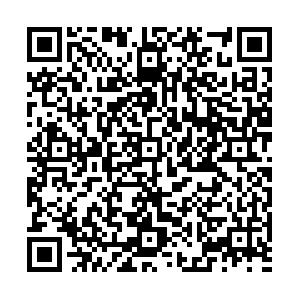# Light mesons and phase structures in μB-T-eB and μI-T-eB spaces

• Light mesons $(\sigma, \pi^0, \pi^\pm)$are investigated in $\mu_B-T-eB$and $\mu_I-T-eB$spaces using a two-flavor NJL model and used to determine chiral symmetry restoration and the pion superfluid phase transition. In $\mu_B-T-eB$space, during the chiral symmetry restoration process, the mass of the pseudo-Goldstone mode $\pi^0$increases, with sudden jumps. At the critical end point, the $\pi^0$meson exhibits a sharp but continuous mass increase, with a sudden mass jump at the Mott transition. In the nearby first order chiral phase transition region, we observe two $\pi^0$mass jumps, one induced by the Mott transition and the other by the quark mass jump. The mass of the Higgs mode σ first decreases and then increases with chiral symmetry restoration, only showing a jump at the first order chiral phase transition. We plot a chiral phase diagram in terms of the change in quark mass, the Mott transition of the pseudo-Goldstone mode $\pi^0$, and the minimum mass of the Higgs mode σ. Owing to explicit breaking of chiral symmetry in the physical case, the chiral restoration phase boundaries on the $\mu_B-T$plane from the order parameter side and meson side are different. The $\pi^0$and σ mass jumps will be helpful to the experimental search for the chiral phase diagram and critical end point. On the $\mu_I-T$plane, the competition between the pion superfluid phase transition and chiral symmetry restoration under magnetic fields is studied in terms of the Goldstone mode $\pi^+$and pseudo-Goldstone mode $\pi^0$. In contrast to the two mass jumps of $\pi^0$in the first order chiral phase transition region, the $\pi^+$meson displays several mass jumps in the chiral crossover region. At the critical end point, $\pi^+$also has sharp but continuous mass change, with a mass jump at the Mott transition. The isospin symmetry is strict, and the pion superfluid phase transition is uniquely determined by the massless Goldstone mode $\pi^+$. The separation of chiral restoration and the pion superfluid phase boundaries is enhanced by the external magnetic field.
••Get Citation
Luyang Li and Shijun Mao. Light mesons and phase structures in μB-T-eB and μI-T-eB spaces[J]. Chinese Physics C. doi: 10.1088/1674-1137/ac7201
Luyang Li and Shijun Mao. Light mesons and phase structures in μB-T-eB and μI-T-eB spaces[J]. Chinese Physics C.Milestone
Article Metric

Article Views(199)
Cited by(0)
Policy on re-use
To reuse of subscription content published by CPC, the users need to request permission from CPC, unless the content was published under an Open Access license which automatically permits that type of reuse.
###### 通讯作者: 陈斌, bchen63@163.com
• 1.

沈阳化工大学材料科学与工程学院 沈阳 110142

Title:
Email:

## Light mesons and phase structures in μB-T-eB and μI-T-eB spaces

###### Corresponding author: Shijun Mao, maoshijun@mail.xjtu.edu.cn, Corresponding author
• 1. Xi’an University of Posts and Telecommunications, Xi’an 710121, China
• 2. Institute of Theoretical Physics, School of Physics, Xi’an Jiaotong University, Xi’an 710049, China

Abstract: Light mesons $(\sigma, \pi^0, \pi^\pm)$are investigated in $\mu_B-T-eB$and $\mu_I-T-eB$spaces using a two-flavor NJL model and used to determine chiral symmetry restoration and the pion superfluid phase transition. In $\mu_B-T-eB$space, during the chiral symmetry restoration process, the mass of the pseudo-Goldstone mode $\pi^0$increases, with sudden jumps. At the critical end point, the $\pi^0$meson exhibits a sharp but continuous mass increase, with a sudden mass jump at the Mott transition. In the nearby first order chiral phase transition region, we observe two $\pi^0$mass jumps, one induced by the Mott transition and the other by the quark mass jump. The mass of the Higgs mode σ first decreases and then increases with chiral symmetry restoration, only showing a jump at the first order chiral phase transition. We plot a chiral phase diagram in terms of the change in quark mass, the Mott transition of the pseudo-Goldstone mode $\pi^0$, and the minimum mass of the Higgs mode σ. Owing to explicit breaking of chiral symmetry in the physical case, the chiral restoration phase boundaries on the $\mu_B-T$plane from the order parameter side and meson side are different. The $\pi^0$and σ mass jumps will be helpful to the experimental search for the chiral phase diagram and critical end point. On the $\mu_I-T$plane, the competition between the pion superfluid phase transition and chiral symmetry restoration under magnetic fields is studied in terms of the Goldstone mode $\pi^+$and pseudo-Goldstone mode $\pi^0$. In contrast to the two mass jumps of $\pi^0$in the first order chiral phase transition region, the $\pi^+$meson displays several mass jumps in the chiral crossover region. At the critical end point, $\pi^+$also has sharp but continuous mass change, with a mass jump at the Mott transition. The isospin symmetry is strict, and the pion superfluid phase transition is uniquely determined by the massless Goldstone mode $\pi^+$. The separation of chiral restoration and the pion superfluid phase boundaries is enhanced by the external magnetic field.

### HTML关注分享DownLoad:  Full-Size Img  PowerPoint